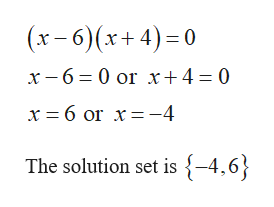# Solve the polynomial inequality and graph the solution set on a real number line. Express the solution set in interval notation.(x-6)(x+ 4)s0Solve the inequality. What is the solution set? Select the correct choice below and, if necessary, fill in the answer box to complete your choiceO A. The solution set is(Simplity your answer. Type your answer in interval notation. Type an exact answer, using radicals as needed Use integers or fractions for any numbers inthe expression.)O B. The solution set is the empty set.Which number line below shows the graph of the solution set?O B.-8O A.-8 -7 6765-2-1-3-7 -6-551-3 -2 -1-5-4OD-8O C.6 7 851-1-3-4-2-5-6-776-3 2 10 12 3-5-6-8 7Click to select your answeraryere to searchWhpT PACK AR Df3fsf610+12insprt scDIIdeletehome\$&73568numlockbackspaceRTUPhoFKLenterCBpauseT shiftaltctrlOI

Question
34 views
check_circle

Step 1

Calculation:

The given quadratic inequality is (x–6)(x+4)≤ 0.

Change the inequality sign by equal sign to form equivalent equation as (x–6)(x+4)=0.

Note that, if the product of two algebraic expressions is zero, then at least one of the factors is equal to zero.

use the note and solve the above equation as follows.help_outlineImage Transcriptionclose(x-6)(x+ 4) 0 x -6 0 or x+ 4 = 0 x 6 or x-4 The solution set is {-4,6} fullscreen
Step 2

The solutions divide the x axis into the intervals as  (–∞,–4), (–4,0), (0,6), and (6,∞).

Find the sign of the polynomial on each interval by selecting test value as follows.

Let the polynomial function be f(x)=(x–6)(x+4).

Obtain the sign of polynomial f(x) at the point x=–5 on the interval (–∞,–4).

Step 3

Obtain the sign of polynomial f(x) at the point x=&nda...

### Want to see the full answer?

See Solution

#### Want to see this answer and more?

Solutions are written by subject experts who are available 24/7. Questions are typically answered within 1 hour.*

See Solution
*Response times may vary by subject and question.
Tagged in

### Equations and In-equations